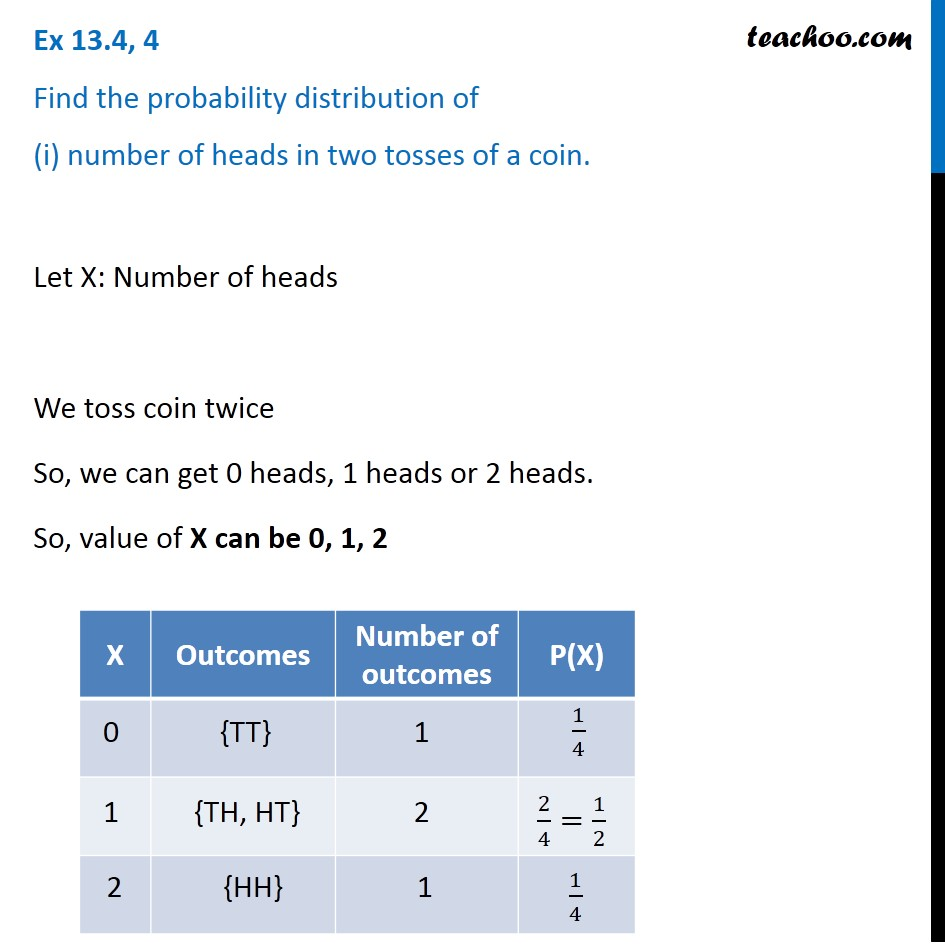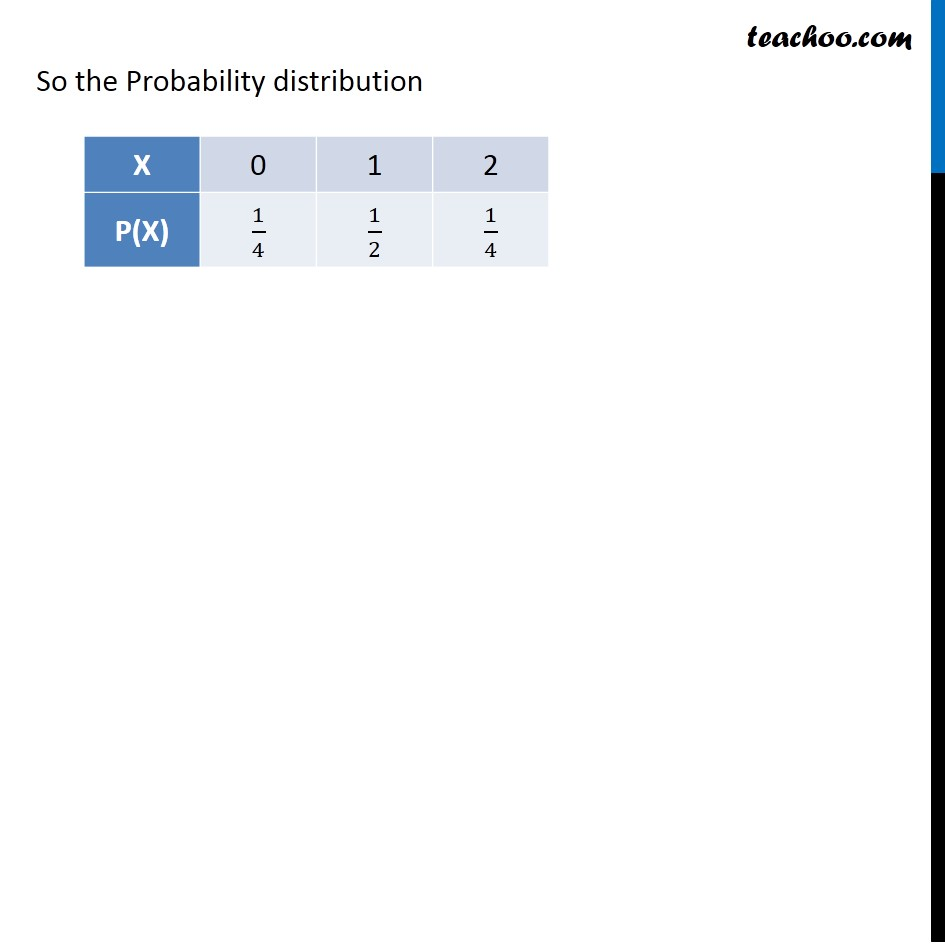Probability Distribution

Chapter 13 Class 12 Probability
Serial order wiseLearn in your speed, with individual attention - Teachoo Maths 1-on-1 Class

### Transcript

Question 4 Find the probability distribution of (i) number of heads in two tosses of a coin. Let X: Number of heads We toss coin twice So, we can get 0 heads, 1 heads or 2 heads. So, value of X can be 0, 1, 2 So the Probability distribution